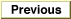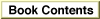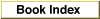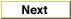# Legacy DocumentImportant: The information in this document is obsolete and should not be used for new development.

Inside Macintosh: Operating System Utilities /

# Chapter 3 - Mathematical and Logical Utilities

This chapter describes a number of utility routines that you can use to perform mathematical and logical operations supported directly by the Macintosh Operating System. In particular, this chapter discusses how you can

• perform low-level logical manipulation of bits and bytes when using a compiler that does not directly support such manipulations
• save disk space by using simple compression and decompression routines
• obtain a pseudorandom number
• perform mathematical operations with two fixed-point data types supported directly by the Operating System
• convert numeric variables of different types

You need to read this chapter only if you need access to any of these features. With the exception of the mathematical operations and conversions, the routines this chapter describes are intended for programmers who occasionally need to access some of these features and do not require that the algorithms used to implement them be sophisticated. For example, if you are developing an advanced mathematical application, the pseudorandom number generator built into the Operating System might be too simplistic to fit your needs. Similarly, if you wish to access individual bits of memory in a time-critical loop, the Operating System routines that perform these operations are probably too slow to be practical.

You do not need any prior knowledge of the Operating System to read this chapter, which begins by describing the building blocks of memory in any operating system: bits, bytes, words, and long words. After subsequent discussions of the built-in compression and decompression routines provided by the Operating System, this chapter illustrates how you can use the Operating System's Mathematical and Logical Utilities. The chapter concludes with a reference to all mathematical and logical routines supported by the Operating System. If you are an experienced programmer, you might be able to skip directly to that section to determine which routine you need.

This chapter does not describe the numeric data types supported by the Standard Apple Numerics Environment (SANE) that the Operating System does not support directly. For more information on such data types, consult the Apple Numerics Manual and Inside Macintosh: PowerPC Numerics.

Chapter Contents
About the Mathematical and Logical Utilities
Bits, Bytes, Words, and Long Words
Bit Manipulation and Logical Operations
Reversed Bit-Numbering
Data Compression
Pseudorandom Number Generation
Fixed-Point Data Types
Angle-Slope Conversion
Using the Mathematical and Logical Utilities
Performing Low-Level Manipulation of Memory
Testing and Manipulating Bits
Performing Logical Operations on Long Words
Extracting a Word From a Long Word
Hardcoding Byte Values
Compressing Data
Obtaining Pseudorandom Numbers
Using Fixed-Point Data Types
Mathematical and Logical Utilities Reference
Data Structures
64-Bit Integer Record
Routines
Testing and Setting Bits
Performing Logical Operations
Getting and Setting Memory Values
Compressing and Decompressing Data
Obtaining a Pseudorandom Number
Converting Between Angle and Slope Values
Multiplying and Dividing Fixed-Point Numbers
Performing Calculations on Fixed-Point Numbers
Converting Among 32-Bit Numeric Types
Converting Between Fixed-Point and Floating-Point Values
Converting Between Fixed-Point and Integral Values
Multiplying 32-bit values
Summary of the Mathematical and Logical Utilities
Pascal Summary
Data Types
Routines
C Summary
Data Types
Routines
Global Variables